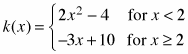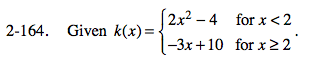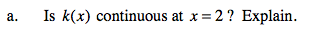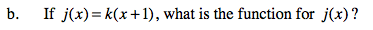### Home > PC > Chapter 2 > Lesson 2.3.7 > Problem2-164

2-164.
1. Given. Try this using the 2-164 eTool (Desmos). Homework Help ✎

1. Is k(x) continuous at x = 2? Explain.

2. If j(x) = k(x + 1), what is the function for j(x)?Substitute x = 2 into each piece of the function.Substitute (x + 1) for every x in k(x). Remember to change the domain as well.

Use the eTool below to complete parts (a) and (b).
Click the link at right for the full version of the eTool: PCT 2-164 HW eTool